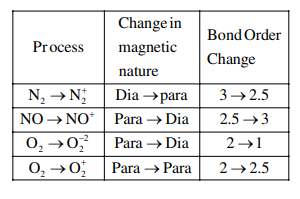# In which of the following processes, the bond order has increased and paramagnetic character has changed to diamagnetic ?`
Question:

In which of the following processes, the bond order has increased and paramagnetic character has changed to diamagnetic ?

1. $\mathrm{N}_{2} \rightarrow \mathrm{N}_{2}^{+}$

2. $\mathrm{NO} \rightarrow \mathrm{NO}^{+}$

3. $\mathrm{O}_{2} \rightarrow \mathrm{O}_{2}^{2-}$

4. $\mathrm{O}_{2} \rightarrow \mathrm{O}_{2}^{+}$

Correct Option: , 2

Solution: